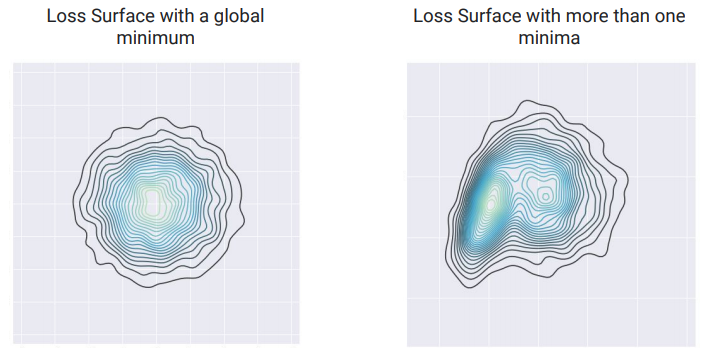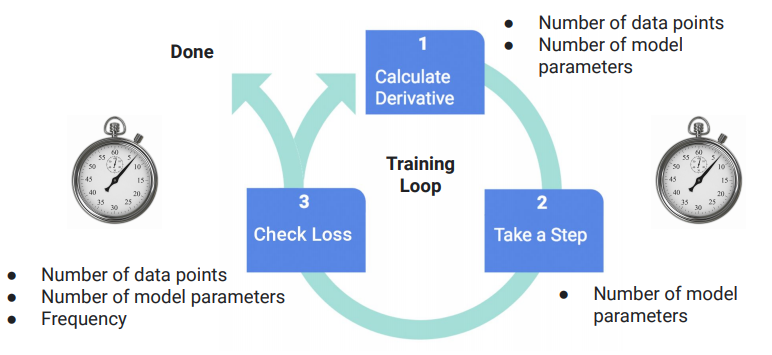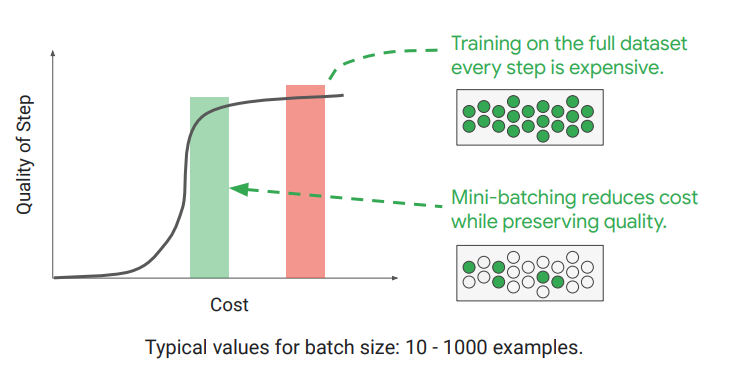DAY 12
0
AI & Data

# 每次重新訓練模型都會改變# 模型訓練實在太慢``````while loss is > Epsilon
derivative = computeDerivative()
for i in range(weights.size):
weights[i] = weights[i] - learning_rate * derivative[i]
if readyToSampleLoss():
loss = sampleLoss()
``````